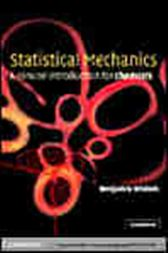Statistical Mechanics

A Concise Introduction for ChemistsUS\$64.00
Statistical mechanics is the theoretical apparatus used to study the properties of macroscopic systems - systems made up of many atoms or molecules - and relates those properties to the system's microscopic constitution. This book is an introduction to statistical mechanics, intended to be used either by advanced undergraduates or by beginning graduate students. The first chapter deals with statistical thermodynamics and aims to quickly derive the most commonly used formulas in the subject. The remainder of the book then illustrates the application of these formulas in traditional areas such as the ideal gas and less traditional areas such as the quantum ideal gas. Highly illustrated with numerous exercises and worked solutions, it provides a concise treatise of statistical mechanics ideal for use on an 8-12 lecture course.

• Cambridge University Press; April 2002
• ISBN: 9780511075001